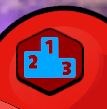The scoring is calculated by taking the letter of each word, getting the corresponding letter value (in table below), and then adding up each letter to get to the value of the word.  You only get this score when you actually beat the level you are on.

If, for example, you play a level and find the following words:

CAT = 3 + 1 + 2
AMAZE = 1 + 4 + 1 + 55 + 1
JET = 52 + 1 + 2
CABBAGE = 3 + 1 + 6 + 6 + 1 + 4 + 1
ABOUT = 1 + 6 + 2 + 3 + 2
RADIO = 2 + 1 + 3 + 1 + 2

This level is word 169 points if you beat the level, or 0 points is you lose.

```Letter Values

'E' = 1
'S' = 1
'I' = 1
'A' = 1
'R' = 2
'N' = 2
'T' = 2
'O' = 2
'L' = 2
'C' = 3
'D' = 3
'U' = 3
'G' = 4
'P' = 4
'M' = 4
'H' = 5
'B' = 6
'Y' = 7
'F' = 8
'V' = 11
'K' = 11
'W' = 12
'Z' = 25
'X' = 41
'J' = 52
'Z' = 55

```